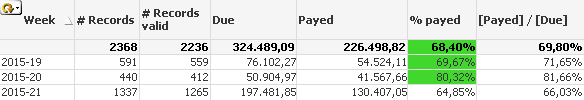# QlikView Scripting

Discussion Board for collaboration on QlikView Scripting.

Announcements

#### Breathe easy -- you now have more time to plan your next steps with Qlik! QlikView 11.2 Extended Support is now valid through December 31, 2020. Click here for more information.

New Contributor II

## Dividing 2 Expressions in a chart: A Different result for the 'same' calculation.

I have an annoying and rather difficult to explain problem.
My data model uses a calendar table (to generate a complete calandar) and it's linked to a data table on one field.

It doesn't link to any other tables in the model.

My 'chart' uses the calendartable as a dimension (by month, week , year), and the values from the data table to calculate the expressions.

Expression "Amount_Open"

=Sum(DataTable_Amount_Due)

Expression "Amounth_Payed)"
=Sum(DataTable_Amount_Payed)

So if I want the % payed

=(Sum(DataTable_Amount_Payed))

/

(Sum(DataTable_Amount_Due))

However

If I calculate essentially the same column using
[Amount_Open] / [Amount_Payed]

I get a difference of several %.

The actual code is a bit more complex  as there is a some basic set analysis in the sums to filter out certain data rows. And the actual Amounth Due And payed is calculated from several fields as well.
However It's the same in both expressions. I don't believe it's a rounding error as even rounding to 0.00000001 doesn't impact the result I get from the combined expression.

Any suggestions on where to look for the problem?

Tags (3)
4 RepliesMVP

## Re: Dividing 2 Expressions in a chart: A Different result for the 'same' calculation.

Would you be able to share a screenshot of the two expressions in a straight table?

New Contributor II

## Re: Dividing 2 Expressions in a chart: A Different result for the 'same' calculation.

Thx for the reply.

Here's a cleaned up test table.And by putting it in a smaller test table...
I just noticed the expression for 'Payed' did not filter out the invalid records, but the expression for % payed did.
My bad, will have to debug in small test tables more often.MVP

## Re: Dividing 2 Expressions in a chart: A Different result for the 'same' calculation.

So is the issue resolved for you now?

New Contributor II

## Re: Dividing 2 Expressions in a chart: A Different result for the 'same' calculation.

Yes, The issue was me not noticing an obvious error because I was "debugging" in a cluttered tableCommunity Browser